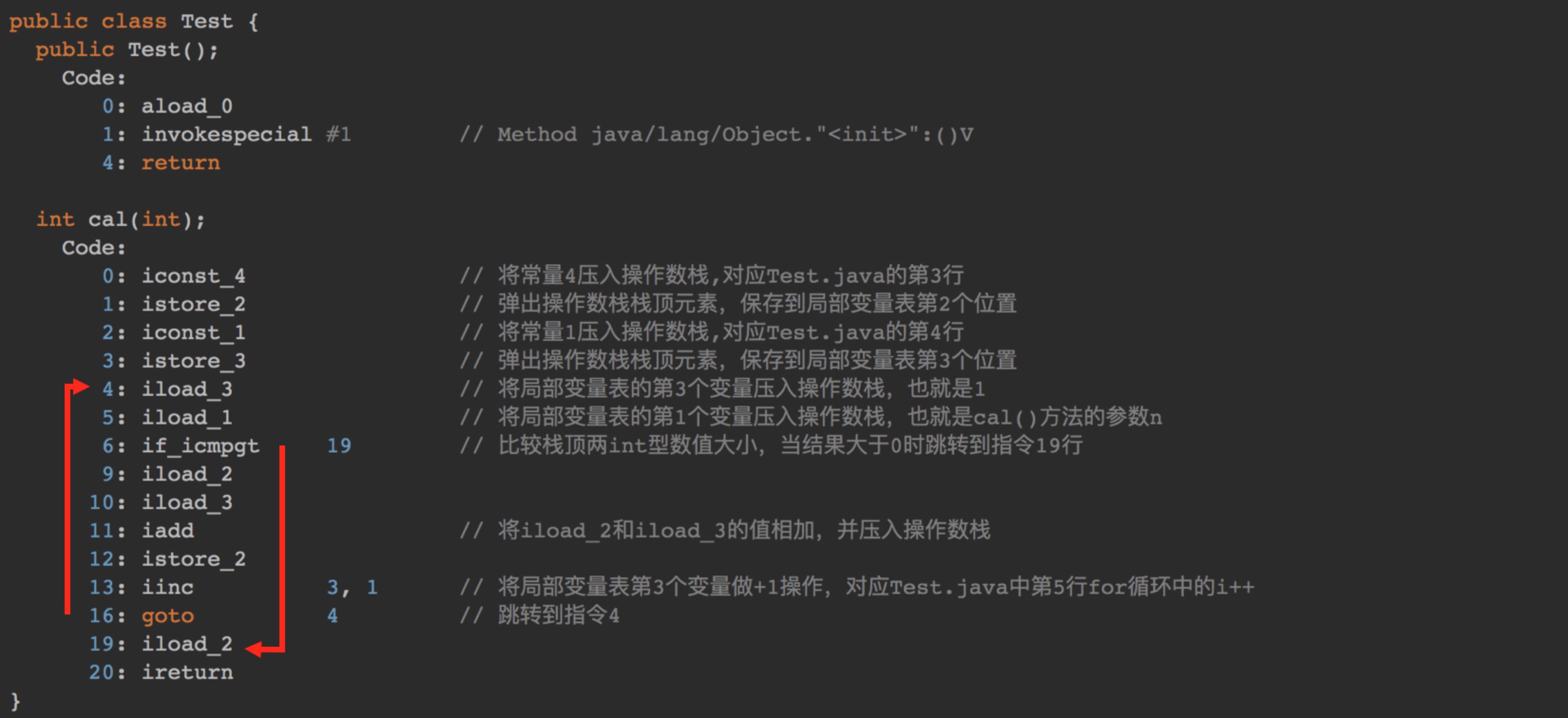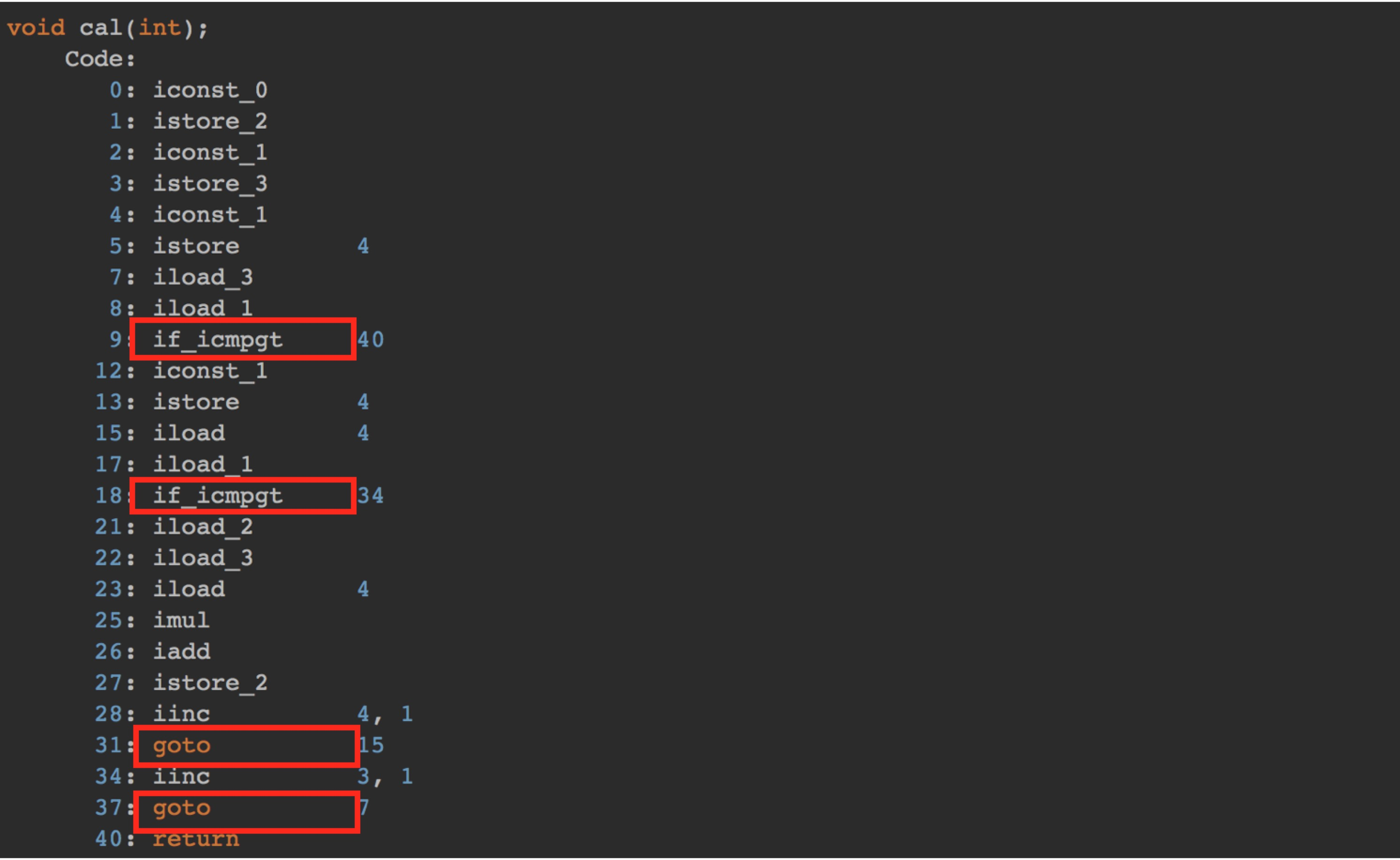# 算法时间复杂度的由来

##### 案例1：
// 计算 1, 2, 3…n 的累加和
public class Test {
int cal(int n) {
int sum = 0;
int i = 1;
for (; i <= n; ++i) {
sum = sum + i;
}
return sum;
}
}


1 测试结果极度依赖测试环境

2 测试结果受数据规模的影响很大

int arr[] = {4, 10, 42, 1, 9};

# 代码复杂度的分析过程

算法的执行时间 = 操作1 + 操作2 + ... + 操作n



javac Test.java    // 编译java代码，生成.class字节码文件
javap -c Test      // 使用javap工具查看字节码指令if_icmpgt 指令判断的是操作数栈顶的两个元素的大小，也就是 i 与 n 的大小，因为从指令 4 到指令 19 一共包含10条指令，所以4~19的指令执行次数为：10 * n * unit_time

4 * unit_time + 10 * n * unit_time
= (10n + 4) * unit_time

##### 案例2
void cal(int n) {
int sum = 0;
int i = 1;
int j = 1;
for (; i <= n; ++i) {
j = 1;
for (; j <= n; ++j) {
sum = sum +  i * j;
}
}
}# 算法时间复杂度表示法

##### 我们可以把这个规律总结成一个公式，注意，大O要登场了
 T(n) = O(f()n)


45n² 高阶项 : 45 * 3 * 3 = 405 占总时间的 95.29%
5n 低阶项 : 5 * 3 = 15
5 常数项 : 5

45n² 高阶项 : 45 * 100 * 100 = 4500 占总时间的 99.98%
5n 低阶项 : 5 * 10 = 50
5 常数项 : 5

• 首先，我们可以忽略常数项，因为随着n的值变得越来越大，常数项最终变得可忽略不计
• 其次，我们可以忽略系数
• 最后，我们只需要考虑高阶项的因子即可，不需要考虑低阶项

# 时间复杂度简单规则：

##### 1 只关注循环执行次数最多的一段代码

int cal(int n) {
int sum = 0;
int i = 1;
for (; i <= n; ++i) {
sum = sum + i;
}
return sum;
}


##### 2 加法法则：总复杂度登记量级最大的那段代码的复杂度

int cal(int n) {
int sum_1 = 0;
int p = 1;
for (; p < 100; ++p) {
sum_1 = sum_1 + p;
}
int sum_2 = 0;
int q = 1;
for (; q < n; ++q) {
sum_2 = sum_2 + q;
}
int sum_3 = 0;
int i = 1;
int j = 1;
for (; i <= n; ++i) {
j = 1;
for (; j <= n; ++j) {
sum_3 = sum_3 +  i * j;
}
}
return sum_1 + sum_2 + sum_3;
}


##### 3 乘法法则：嵌套代码的复杂度等于嵌套内外代码复杂度的乘积

int cal(int n) {
int ret = 0;
int i = 1;
for (; i < n; ++i) {
ret = ret + f(i);
}
}
int f(int n) {
int sum = 0;
int i = 1;
for (; i < n; ++i) {
sum = sum + i;
}
return sum;
}


T(n) = T1(n) * T2(n) = O(n*n) = O(n²)。

# 总结

#### 更多文章可以扫描二维码，关注算法公众号09-273万+06-21613
05-301万+
01-19235
01-29474
09-2028万+
08-142万+
11-27203
11-27694
©️2020 CSDN 皮肤主题: 大白 设计师:CSDN官方博客